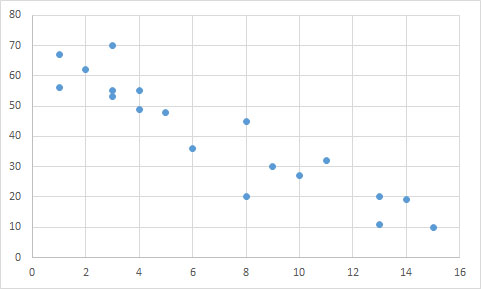# High School Math : Representing Data

## Example Questions

### Example Question #1 : Basic Statistics

Interquartile range

Mode

Mean

Range

Median

Median

Explanation:

A box and whisker plot separates the data into quartiles so that each quartile has an equal number of data points. The box indicates the interquartile range, that is, the top line of the box is the third quartile and the bottom line of the box is the second quartile. The line separating the second and third quartiles indicates the median. The lines outside of the box indicate the outer-quartiles (first and fourth).

### Example Question #2 : How To Interpret Dotplots

Based on the scatter plot below, is there a correlation between theandvariables? If so, describe the correlation.Yes; negative linear relationship

Yes; negative exponential relationship

No; there is no correlation

Yes; positive linear relationship